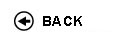Politecnico di Torino
16ACFLZ, 16ACFLN, 16ACFLP, 16ACFLS, 16ACFLX, 16ACFMA, 16ACFMB, 16ACFMC, 16ACFMH, 16ACFMK, 16ACFMN, 16ACFMO, 16ACFMQ, 16ACFNL, 16ACFNM, 16ACFNX, 16ACFOA, 16ACFOD, 16ACFPC, 16ACFPI, 16ACFPL, 16ACFQR
Mathematical analysis I
1st degree and Bachelor-level of the Bologna process in Aerospace Engineering - Torino
1st degree and Bachelor-level of the Bologna process in Automotive Engineering - Torino
1st degree and Bachelor-level of the Bologna process in Electronic And Communications Engineering - Torino
Espandi...
 Teacher Status SSD Les Ex Lab Tut Years teaching Adami Riccardo O2 MAT/05 60 40 0 0 8 Berchio Elvise A2 MAT/05 60 40 0 0 8 Camporesi RobertoAC MAT/05 60 40 0 0 10 Ceragioli Francesca MariaA2 MAT/05 60 40 0 0 10 Chiado' Piat ValeriaO2 MAT/05 60 40 0 0 18 Codegone Marco60 40 0 0 14 Grillo Alfio A2 MAT/07 60 40 0 0 8 Mazzi LuisaAC MAT/05 60 40 0 0 21 Nicola Fabio O2 MAT/05 60 40 0 0 10 Pandolfi Luciano60 40 0 0 12 Pandolfi Luciano60 40 0 0 12 Tabacco Anita MariaPO MAT/05 60 40 0 0 22 Tilli PaoloPO MAT/05 60 40 0 0 9 Zanini ChiaraA2 MAT/05 60 40 0 0 7 Zanini ChiaraA2 MAT/05 60 40 0 0 7 Cortese Paolo60 40 0 0 12 Mercadante Silvio 60 40 0 0 3 Quelali Gutierrez Guillermo Gonzalo 60 40 0 0 5
 SSD CFU Activities Area context MAT/05 10 A - Di base Matematica, informatica e statistica
Esclusioni:
04KWQ
 Subject fundamentals This course represents a bridge between high school and university. The main goal of the course is to provide the students with tools to follow the concatenation of simple logical arguments, and to introduce the fundamentals of differential and integral calculus for functions of one variable, with applications to ordinary differential equations of the first and the second order. Expected learning outcomes Ability to follow a chain of logical arguments. Knowledge of the fundamentals of differential and integral calculus for functions of one variable. Learning of an adequate computational ability. Prerequisites / Assumed knowledge Numerical sets, equations and inequalities, analytic geometry and trigonometry. Elementary functions and their first properties. Contents Preliminaries: sets, operations with sets and logical symbols. Numerical sets, maxima, minima and extrema. The completeness property of real numbers and its consequences. Functions: surjectivity and injectivity; composition of functions, inverse functions. Functions of one real variable: elementary functions, monotone functions and inverse functions. (About 15 hours) Limits and continuity: limits of functions and sequences; continuity. Theorems on limits: uniqueness of the limit, sign-preserving property and local boundedness, comparison theorems. Limits of monotone functions. Algebra of limits. Indeterminate forms. Local comparison of functions. Landau symbols. Infinite and infinitesimal functions. Order of an infinity and of an infinitesimal, principal part with respect to a test function. Asymptotes. The number e. Fundamental trigonometric and exponential limits. Continuous functions over an interval: existence of zeros and of maxima and minima. (About 24 hours) Derivatives: geometrical and physical meaning. Computation of derivatives. Derivatives of elementary functions. Derivatives and continuity. Non-differentiability points, extremal and critical points. Fermat Theorem. Functions differentiable over an interval and fundamental theorems of differential calculus (Rolle and Lagrange) and their consequences. De L'H�pital rule. Taylor formula and fundamental Maclaurin expansions. Use of Taylor expansions in the local analysis of functions: comparison, extrema and convexity. Applications to the study of the graph of a function. (About 23 hours) Primitives and their computation; primitives of rational functions. Indefinite integral. The definite integral of a piecewise continuous function. Properties of the integral. Integral mean, the mean value theorem and the Fundamental Theorem of Calculus. Relations between definite integrals and primitives. Improper integrals: definitions and convergence tests. (About 21 hours) Complex numbers and differential equations: algebraic and trigonometric form of a complex number. Real and imaginary parts, modulus and argument. Roots of complex numbers; the Fundamental Theorem of Algebra. The exponential of a complex number and the Euler formula. Ordinary differential equations: the Cauchy problem. First order ordinary differential equations, linear or with separable variables. Second order linear differential equations with constant coefficients. (About 17 hours) Delivery modes 60 hours of theoretical classes and 40 hours of exercise classes. Texts, readings, handouts and other learning resources C. Canuto, A. Tabacco. Mathematical Analysis I. Springer-Verlag UTX, 2015. Additional material will be provided by the teacher and will be available in the Internet personal page of the student. Assessment and grading criteria The exam consists of a test, a written part and, when required, of an oral part. The test (on a computer, one hour long) consists of 20 multiple-choice questions, also of theoretical type. Each question is worth one point, and the maximum achievable score is 20. If the test score is less than 12, then the exam is failed, otherwise the student proceeds with the written part of the exam (consisting of exercises and theoretical questions, one hour long). The written part is worth 13 points. If the score achieved is less than 5, then the exam is failed, otherwise the sum of the scores of the test and the written part is the final score of the exam, unless an oral part is required by the teacher (or by the student, provided that the final score is at least 18/30). In this case the final score will be based also on the oral part. Programma definitivo per l'A.A.2016/17